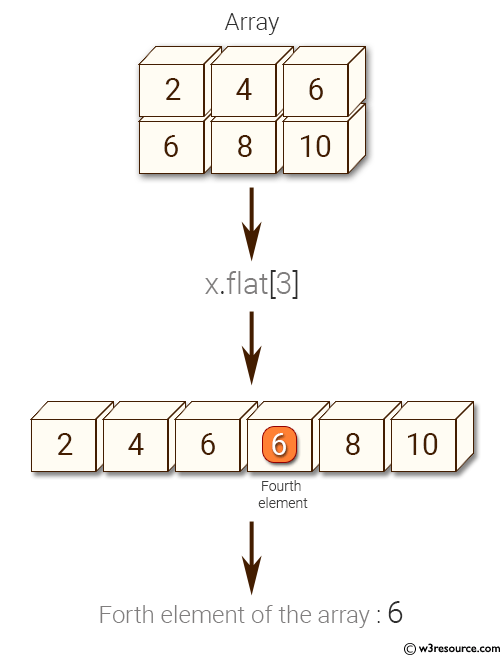﻿ NumPy: Find the 4th element of a specified array - w3resource# NumPy: Find the 4th element of a specified array

## NumPy: Array Object Exercise-50 with Solution

Write a NumPy program to find the 4th element of a specified array.

Pictorial Presentation:Sample Solution:

Python Code :

``````import numpy as np
x = np.array([[2, 4, 6], [6, 8, 10]], np.int32)
print(x)
e1 = x.flat
print("Forth e1ement of the array:")
print(e1)
```
```

Sample Output:

```[[ 2  4  6]
[ 6  8 10]]
Forth e1ement of the array:
6
```

Python Code Editor:

Have another way to solve this solution? Contribute your code (and comments) through Disqus.

What is the difficulty level of this exercise?

Test your Python skills with w3resource's quiz

﻿

## Python: Tips of the Day

List comprehension:

```>>> m = [x ** 2 for x in range(5)]
>>> m
[0, 1, 4, 9, 16]
```